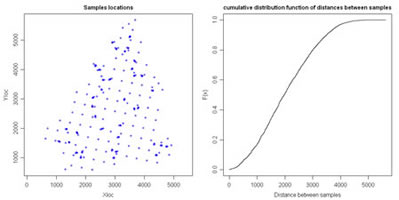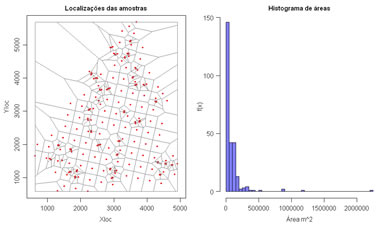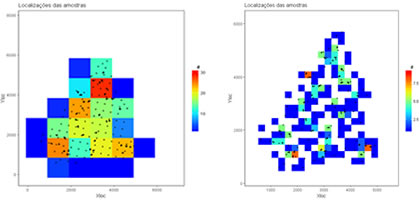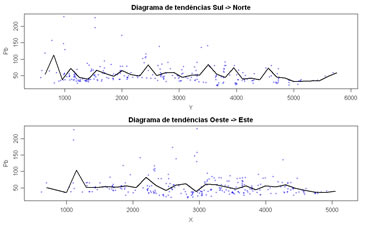## 6. Declustering and trend analysis

 6.1 Distance betwen samples (download) Compute distances between all pairs of samples and plot distances in a cumulative histogram. Variables to customize:  `varcolXY <- c(1,2) # index of the GTD frame X and Y coordinatessizePOINTS <- 1  # size of the sample symbols of the left plottitleGRAPH <- "Samples locations" # global title of the left plot`6.2 Compute Vononoi Polygons (download) Compute Voronoi polygons and compute declustered basic statistics. An histogram of the Voronoi polygon areas are displayed at right. Variables to customize: `varcolXY <- c(1,2) # index of the GTD frame X and Y coordinatesvarlistindex <- c(5,6,7,8,9,10,11) # index of the GTD frame Qualitative Variables to recompute declustered basic statisticstitleGRAPH <- "Representação em planta das amostras de solos" # global title of the left plotndecimals <- 3 # number of decimal places`6.3 Cell declustering (download) Overlap a set of samples with a mesh of cells and compute average values of several variables within each cell. After, compute global declustered basic statistics. Variables to customize: `varcolXY <- c(1,2) # index of the GTD frame X and Y coordinatesvarlistindex <- c(5,6,7,8,9,10,11) # index of the GTD frame Qualitative Variables to recompute declustered basic statisticstitleGRAPH <- "Localizações das amostras" # global title of the left plotndecimals <- 3 # number of decimal placescellsizex <- 250 # size of the cells in X directioncellsizey <- 250 # size of the cells in Y direction`6.4 Spatial trend analysis in X and Y directions (download) Perform a trend analysis of a quantitative variable along X and Y directions. Variables to customize: `varcolXY <- c(1,2) # index of the GTD frame X and Y coordinatesvarlistindex <- 10 # index of the GTD quantitative variable to trend analysisndecimals <- 3 # number of decimal placescellsizex <- 150 # size of the cells in X directioncellsizey <- 150 # size of the cells in Y directionsizePOINTS <- 2 # size of the symbols to plot`